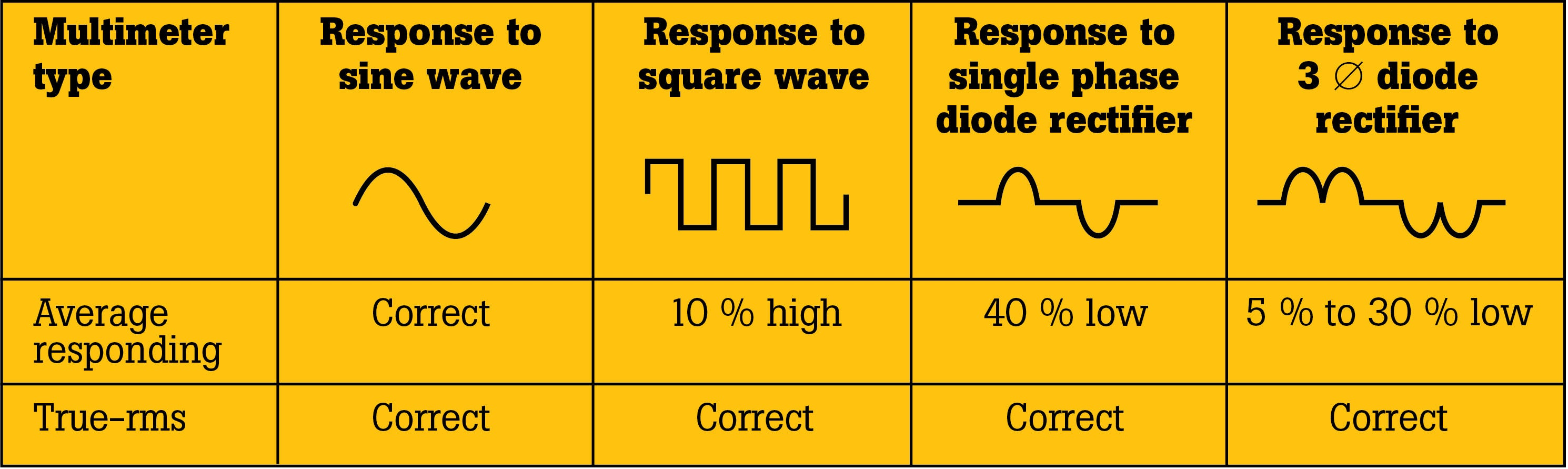## CATEGORIESWhat is true-RMS

What is true-RMS?
True-RMS (root mean squar)  holds significant importance in electrical measurements. It refers to a method of accurately measuring alternating current (AC) or AC voltage. There are three primary tools that can be used for this purpose:

1. True-RMS digital multimeters (or clamp meters)
2. Average-responding digital multimeters (or clamp meters)
3. Oscilloscopes

Among these options, the first two tools are commonly employed and are capable of accurately measuring standard sinusoidal waveforms. However, the true-RMS meter is widely preferred due to its ability to accurately measure both sinusoidal and non-sinusoidal AC waveforms.

To comprehend the calculation of the root mean square (RMS), it is essential to understand that RMS determines the equivalent direct current (DC) value of an AC waveform. It quantifies the "effective" or DC heating value of any AC wave shape. Here is a breakdown of the tools used for measurement:

• An average-responding meter uses averaging mathematical formulas to accurately measure pure sinusoidal waves. However, its accuracy is uncertain when measuring non-sinusoidal waves.

• On the other hand, a more sophisticated true-RMS meter can accurately measure both pure waves and the more complex non-sinusoidal waves. Nonlinear loads, such as variable speed drives or computers, can distort waveforms. When an averaging meter attempts to measure distorted waves, it can be up to 40% low or 10% high in its calculations.

RMS voltage refers to the voltage value obtained through the true-RMS measurement method. To measure true-RMS accurately, it is crucial to use appropriate meters. The demand for true-RMS meters has grown significantly in recent years due to the prevalence of non-sinusoidal waves in various circuits.

Why is True-RMS So Important?

When measuring AC voltage or current signals that are not pure sinusoidal waves, the use of a true-RMS meter becomes crucial due to the prevalence of nonsinusoidal waveforms in most electronic loads. Here's why true-RMS is so important:

1. Impact of Waveform Shape on Readings: The shape of the waveform, whether pure sinusoidal or nonsinusoidal, significantly affects the accuracy of readings obtained with different types of multimeters.

2. Accuracy of Average Responding Meters: Average responding meters use averaging formulas to measure pure sinusoidal waves accurately. However, when measuring nonsinusoidal waves, the accuracy of these meters becomes uncertain. The average responding meter assumes a sinusoidal wave and calculates the average of the highest and lowest parts, multiplied by a conversion factor. In the case of distorted or uneven waveforms, this average measurement can be up to 40% low or 10% high, depending on the waveform.

3. Scenarios Requiring True-RMS Measurement: To ensure accurate measurements, a true-RMS meter is necessary in situations involving nonsinusoidal waves, such as:

• Variable-frequency drives (VFDs)
• Electronic ballasts
• Computers
• HVAC control systems
• Solid-state environments
1. Functioning of True-RMS Meters: True-RMS meters accurately measure both imperfect, nonsinusoidal waves and perfect, sinusoidal waves. They utilize mathematical equations to convert measurements and provide accurate readings for both types of waveforms. The calculation of root-mean-square (RMS) is used to determine the equivalent DC value of an AC waveform.

2. True-RMS Application in HVAC: In HVAC systems, where energy-efficient modulating systems are increasingly common, true-RMS meters are essential. When servicing these units, accurate measurements are crucial, considering the computerized controls, solid-state variable frequency drives (VFDs), and VFD-controlled motors. True-RMS measurements enable accurate troubleshooting and analysis of issues in such systems.

In summary, the importance of true-RMS lies in its ability to accurately measure both sinusoidal and nonsinusoidal waveforms, ensuring reliable readings in various electronic load scenarios and applications like HVAC systems.